Courses

# Test: Flow of Compressible Fluids - 1

## 10 Questions MCQ Test Topicwise Question Bank for Mechanical Engineering | Test: Flow of Compressible Fluids - 1

Description
This mock test of Test: Flow of Compressible Fluids - 1 for Mechanical Engineering helps you for every Mechanical Engineering entrance exam. This contains 10 Multiple Choice Questions for Mechanical Engineering Test: Flow of Compressible Fluids - 1 (mcq) to study with solutions a complete question bank. The solved questions answers in this Test: Flow of Compressible Fluids - 1 quiz give you a good mix of easy questions and tough questions. Mechanical Engineering students definitely take this Test: Flow of Compressible Fluids - 1 exercise for a better result in the exam. You can find other Test: Flow of Compressible Fluids - 1 extra questions, long questions & short questions for Mechanical Engineering on EduRev as well by searching above.
QUESTION: 1

### At normal atompsheric conditions, the ratio of the speed of sound in water to that in air is about

Solution:

The sonic velocity in air at 15°C is about 340 m/s and that in water at the same temperature is about 1440 m/s.

QUESTION: 2

### The velocity of sound is largest in

Solution:

Sonic velocity is higher in flow mediums with high bulk modulus and low density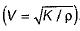QUESTION: 3

### The area velocity relationship for compressible fluid flow is

Solution:

The continuity equation in differential form is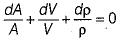The differential form for energy in isentropic flow is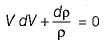From the above two equations,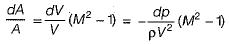QUESTION: 4

The speed of sound in oxygen at a pressure of 100 kPa (absolute) and 27°C is (Take R = 260 J/kg K and K = 1.4)

Solution: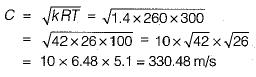QUESTION: 5

An airplane is flying at an altitude where the pressure is 20 kPa (absolute) and the density is 0.28 kg/m3. If the plane has attained a mach number of 1,8, what is the speed of plane in km/hr?

Solution:

Velocity of sound,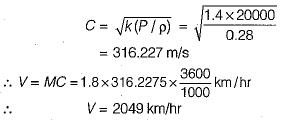QUESTION: 6

A rocket is found to have a speed of 2160 km/hr in air at a temperature of -40°C. What is approximate Mach angle? [Take R = 287 J/kg K and K = 1.4]

Solution: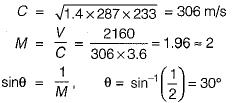QUESTION: 7

Match List-1 (phenomena) with List-il (causes) and select the correct answer using the codes given below the lists: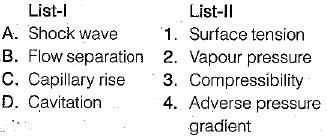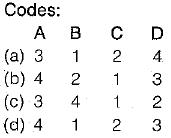Solution:
QUESTION: 8

The prime parameter causing change of state in a Fanno flow is

Solution:

In Fanno Line Flow two assumptions are made
1. No friction
2. During the flow, no heat transfer is taking place.

QUESTION: 9

Acoustic velocity in an elastic gaseous medium is proportional to

Solution: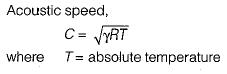QUESTION: 10

In a normal shock wave in one-dimensional flow

Solution: